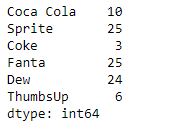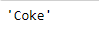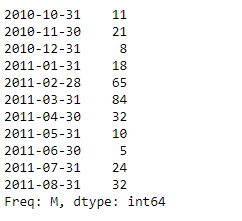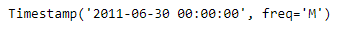# Python | Pandas Series.idxmin()

Pandas series is a One-dimensional ndarray with axis labels. The labels need not be unique but must be a hashable type. The object supports both integer- and label-based indexing and provides a host of methods for performing operations involving the index.

Pandas` Series.idxmin()` function return the row label of the minimum value. If multiple values equal the minimum, the first row label with that value is returned.

Syntax: Series.idxmin(axis=0, skipna=True, *args, **kwargs)

Parameter :
skipna : Exclude NA/null values. If the entire Series is NA, the result will be NA.
axis : For compatibility with DataFrame.idxmin. Redundant for application on Series.

Returns : idxmin : Index of minimum of values.

Example #1: Use `Series.idxmin()` function to find the index label corresponding to the minimum value in the given series object.

 `# importing pandas as pd ` `import` `pandas as pd ` ` `  `# Creating the Series ` `sr ``=` `pd.Series([``10``, ``25``, ``3``, ``25``, ``24``, ``6``]) ` ` `  `# Create the Index ` `index_ ``=` `[``'Coca Cola'``, ``'Sprite'``, ``'Coke'``, ``'Fanta'``, ``'Dew'``, ``'ThumbsUp'``] ` ` `  `# set the index ` `sr.index ``=` `index_ ` ` `  `# Print the series ` `print``(sr) `

Output :Now we will use `Series.idxmin()` function to find the index label corresponding to the minimum value in the series.

 `# return index label of the  ` `# minimum value in the series ` `result ``=` `sr.idxmin() ` ` `  `# Print the result ` `print``(result) `

Output :As we can see in the output, the `Series.idxmin()` function has returned the index label of the minimum element in the given series object.

Example #2 : Use `Series.idxmin()` function to find the index label corresponding to the minimum value in the given series object.

 `# importing pandas as pd ` `import` `pandas as pd ` ` `  `# Creating the Series ` `sr ``=` `pd.Series([``11``, ``21``, ``8``, ``18``, ``65``, ``84``, ``32``, ``10``, ``5``, ``24``, ``32``]) ` ` `  `# Create the Index ` `index_ ``=` `pd.date_range(``'2010-10-09'``, periods ``=` `11``, freq ``=``'M'``) ` ` `  `# set the index ` `sr.index ``=` `index_ ` ` `  `# Print the series ` `print``(sr) `

Output :Now we will use `Series.idxmin()` function to find the index label corresponding to the minimum value in the series.

 `# return index label of the  ` `# minimum value in the series ` `result ``=` `sr.idxmin() ` ` `  `# Print the result ` `print``(result) `

Output :As we can see in the output, the `Series.idxmin()` function has returned the index label of the minimum element in the given series object.

My Personal Notes arrow_drop_upCheck out this Author's contributed articles.

If you like GeeksforGeeks and would like to contribute, you can also write an article using contribute.geeksforgeeks.org or mail your article to contribute@geeksforgeeks.org. See your article appearing on the GeeksforGeeks main page and help other Geeks.

Please Improve this article if you find anything incorrect by clicking on the "Improve Article" button below.

Article Tags :

Be the First to upvote.

Please write to us at contribute@geeksforgeeks.org to report any issue with the above content.